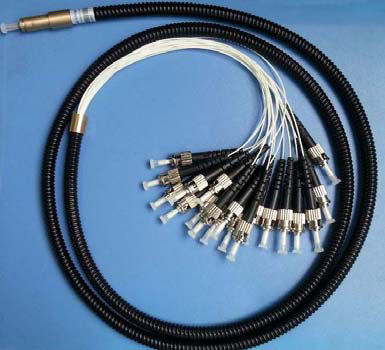### 当前位置：网站首页 / 产品中心

 光纤束产品特点 产品应用 高功率转换效率 高功率全光纤激光器 波长不敏感 高功率光纤放大器 高工作功率 医疗应用 可客户定制 军事应用
 指 标 参数 单位 光纤数 2, 3, 4, 7, 19, 37, 61 转换效率 典型值 % 98 工作功率 最大值 W 5 工作温度 °C -50 to +75 储存温度 °C -50 to +85

 外形图示意图订购信息 F O B 0 0 0 0 0 光纤数量 1=2-Fiber 2=3-Fiber 3=4-Fiber 4=7-Fiber 5=19-Fiber 6=37-Fiber 7=61-Fiber S=Specify 光纤类型 1=105/125μm (NA=0.22) 2=62.5/125μm 3=50/125μm 4=Plastic fiber 5=G625 or Equivalent S=Specify 尾纤长度 1=1.0m 2=1.5m S=Specify 散出长度 1=15cm 2=25cm 3=35cm S=Specify 连接器 0=None 1=FC/PC 2=ST 3=ST/PC S=Specify

2. 所有指标都是不含连接器，如有变更恕不提前通知。